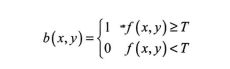﻿ 基于Matlab图像处理的公路裂缝检测实现_C 语言_脚本之家

# 基于Matlab图像处理的公路裂缝检测实现

更新时间：2022年02月23日 11:52:15   作者：紫极神光

## 一、简介

### 2 理论基础

2.1 图像灰度化（1）分量值（2）最大值（3）平均值（4）加权平均值

F.(i.j)=0.299R(i，j)+0.587G(i，j)+0.114*B(i，j)2.2 图像滤波

(1)定位

(2)计算

(3)赋值2.3 图像增强

2.4 图像二值化(1)全局阈值是最常见的阈值计算方法，它一般以图像的直方图或灰度空间分布为基础来确定一个阈值，进而实现灰度图像的二值化。特别是当图像的灰度直方图分布呈双峰时，全局阈值法可以明显地将目标和背景分量，得到较为理想的图像分割效果。但裂缝图像一般具有光照不均匀、噪声干扰等特点，其灰度直方图往往不会呈双峰分布，因此全局阈值分割方法效果较差。

(2)基本自适应阀值是一种比较基础的图像自适应分割方法，它一般以图像像素自身及其邻域灰度变化的特征为基础进行阈值分割，进而实现灰度图像的二值化。该方法充分考虑了每个像素邻域的特征，所以一般能更好地突出目标和背景的边界。

## 二、部分源代码

```    clc;
close all;
clear all;
%% 读取图片
%% 判断图像格式及调整图像尺寸、直方图均衡化
if(ndims(I)==3)%ndims是求数组维数的函数
img=rgb2gray(I);
img=double(img);%转换为双精度
else
img=double(I);%转换为双精度
end
if(max(size(img))>1024)
scale=1/8;  %图像调整为原图尺寸的1/8
I1 = imresize(img, scale);%缩放处理
else
I1=img;
end
[m,n]=size(I1);
f_size=max(m,n);
%% 去噪、锐化
[Slog f2]=frequence_get(I1,f_size);
%% 陷波滤波 去除周期性噪声
% % 用陷波滤波模板修改原图傅里叶频谱，滤除高频周期噪声
% % 构造陷波滤波器模板
I_spec=I1;
I_spec=im2uint8(mat2gray(I_spec));%矩阵归一化，把图像数据类型转换为无符号的8位整形
I_spec(f_size,f_size)=0;
Spectrum=fft2(uint8(I_spec));%2维离散傅里叶变换
Spec=abs(Spectrum);   %幅度谱
phi=angle(Spectrum);  %相位谱
Spec=fftshift(Spec);
Spec1=Spec;
Spec1(vex)=0;
Spec2=ifftshift(Spec1);
CJ=Spec2.*exp(i*phi);  %重建傅里叶频谱
Re_fft2=ifft2(CJ);     %反傅里叶变换，还原图像
Restore=uint8(real(Re_fft2));
%% 对还原图像维纳滤波
% %    维纳滤波
K=wiener2(Restore(1:m,1:n),[3 3]);%维纳滤波（wiener filtering) 一种基于最小均方误差准则、对平稳过程的最优估计器。这种滤波器的输出与期望输出之间的均方误差为最小，因此，它是一个最佳滤波系统。它可用于提取被平稳噪声所污染的信号。
[result,threshold1]=edge(K,'sobel',0.07);% 若原图没有进行均衡化，阈值用0.07，sobel算子边缘检测裂缝
[BW,thresh1]=bwfilter(K,result);%二值化滤波器去干扰
figure,
subplot(151);imshow(I1,[]);title('原始图像');
subplot(152);imshow(Restore(1:m,1:n),[]);title('反傅里叶变换图像');
subplot(153);imshow(K,[]);title('维纳滤波降噪');
subplot(154);imshow(result,[]);title('裂纹检测');
subplot(155);imshow(BW);title('二值化滤波器去干扰');
% %     形态学操作
Se0=strel('line',3,0);%创建一个相对于邻域中心对称的线性结构元素
Se90=strel('line',3,90);
bw_dialte=imdilate(BW,[Se0 Se90]);%膨胀
bw_fill=imfill(bw_dialte,'holes');%填充
bw1=bwmorph(bw_fill,'thin',Inf);%细化
bw2=bwmorph(bw1,'spur',3);  %去毛刺
bw3=bwareaopen(bw2,5);%移除小目标
figure,
subplot(151);imshow(bw_dialte);title('膨胀');
subplot(152);imshow(bw_fill);title('填充');
subplot(153);imshow(bw1);title('细化');
subplot(154);imshow(bw2);title('去毛刺');
subplot(155);imshow(bw3);title('移除小目标');
```

## 三、运行结果